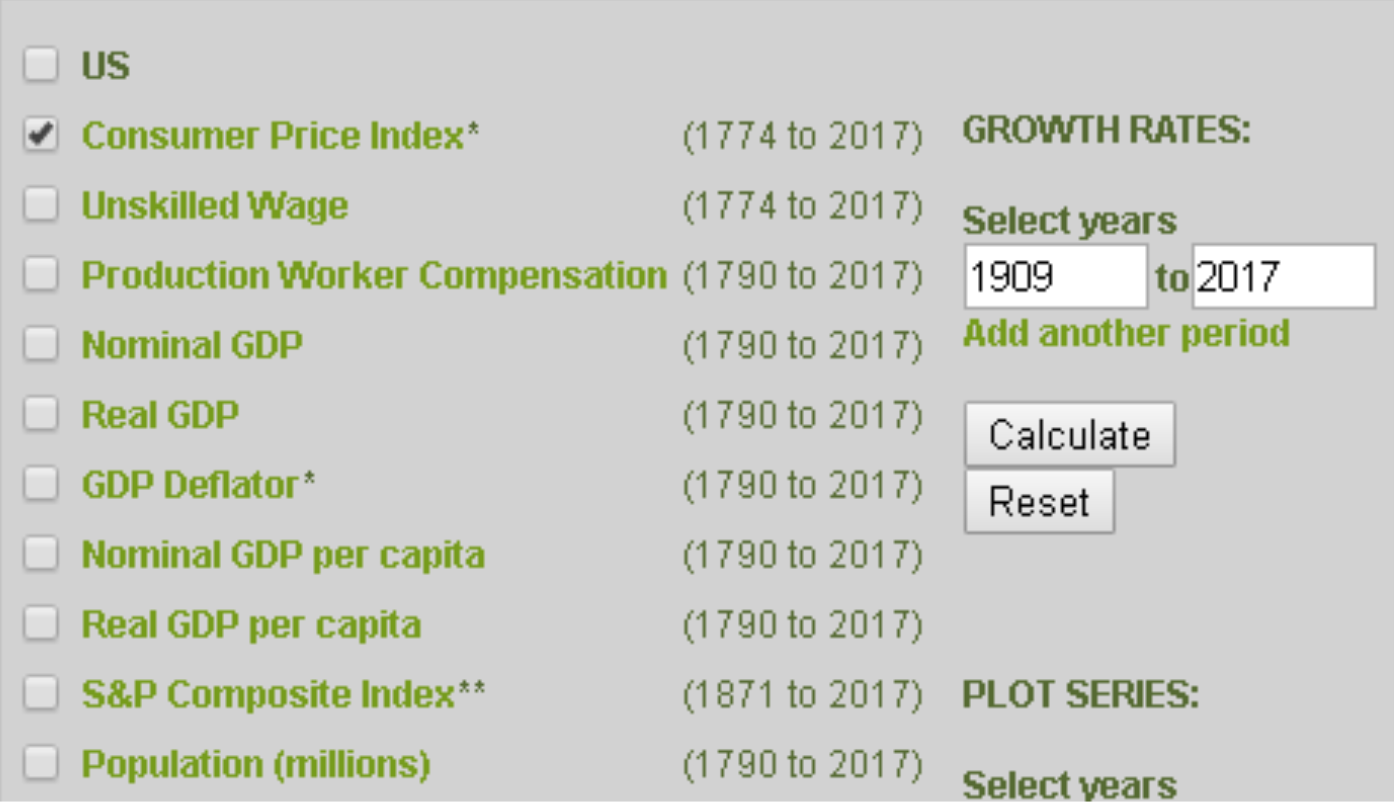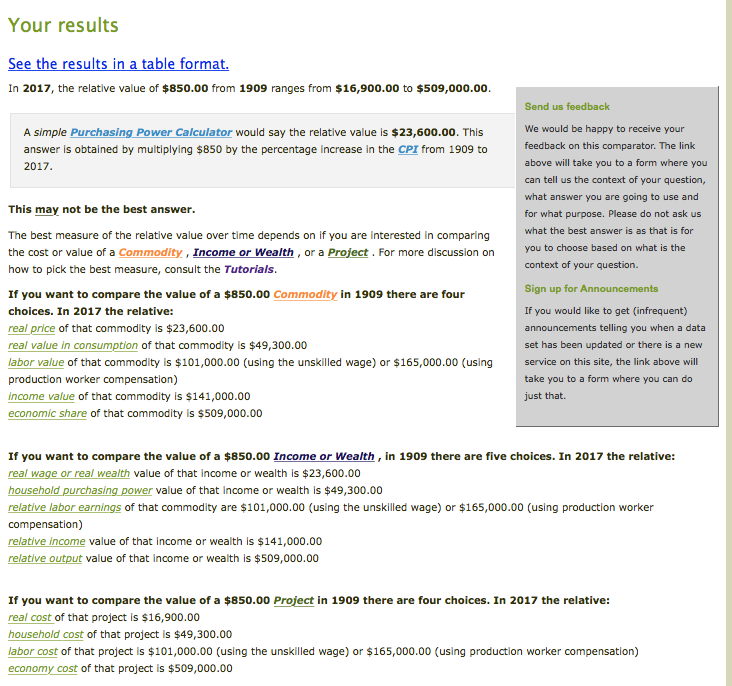### Tutorial 1: How To Find Today’s Worth of a Money Value from the Past

Joanna Short, Augustana College

There are several reasons to convert nominal (current-year) values into real values (the value adjusted for the change in money values over time, e.g. inflation). One is simply to gain a sense of what a money value in the past is “worth” today.

For example, when first introduced in 1909, a Model T Ford (Touring Model) cost \$850 (The Model T Touring Club International). That doesn’t seem like much for a new car, but in general, prices were much lower back then. If we could travel back in time, many, perhaps most, of the goods and services exchanged in 1909 would seem very cheap to us—inflation has been common between 1909 and today. Before you get nostalgic for the “good old days,” wages and earnings were a lot lower, too! Thus, \$850 probably represented a lot of money in 1909. The question remains: how much was it?Let’s suppose that \$850 grew at the same rate as average consumer prices from 1909 to 2017. The U.S. Consumer Price Index (CPI) measures the average cost in urban areas for a basket of consumer goods. Over time, it is used as a measure of inflation. Using the Annualized Growth Rates comparator, check on the U.S. Consumer Price Index and select the years from 1909 to 2017, as shown below. Click on the calculate button, and you’ll obtain an annualized growth rate over that time period of 3.13%. This means that on average, consumer prices increased by 3.13% per year over that time period. Of course, in some years prices increased by a higher percentage and in other years a lower percentage.

We can roughly estimate the value of a Model T today by adjusting for inflation. At an average growth rate of 3.13% per year, over 108 years, we can calculate a present value of \$850 as:

\$850 x (1.0313)108
=\$850 x 27.90
= \$23,715

Thus, we might consider \$850 in 1909 to be the equivalent of about \$23,715 in current dollars, reflecting the inflation that has occurred between 1909 and 2017. Better yet, we can let the calculators do more of this work for us. Using the Relative Values-US \$ comparator, we can simply input an initial year (1909), initial value (\$850) and desired year (2017). The results page, shown below, offers a very wide range of answers, from \$16,900 to \$509,000(!). The results offer some guidance, though, on which of these may be most appropriate for the question at hand.

Notice that one of the results is very close to the one we obtained, \$23,600 reported as the real price of a commodity or the real wage/wealth for an income or wealth value. For this particular result, the calculator used the growth in the CPI, as we did, to calculate the answer. The other results are calculated using other measures of inflation over time, such as the growth in the GDP deflator (another price index), or the growth in unskilled wages. Run your cursor over each result, and a pop-up defines the deflator used for that result. The “correct” measure of value depends mainly on whether the object of interest is considered a commodity, income or wealth, or a project, such as a construction project. Since a Model T is a consumer good, or a commodity, one of the commodity values (real price, real value in consumption, labor value, or income value) would likely best measure the “cost” of the car in today’s terms. However, in other situations, we might want to use another measure of inflation. See Tutorial 4, or Explaining the Measures of Worth for more on which result is most appropriate according to the subject at hand.Finally, we can get a sense of how much a Model T cost by comparing \$850 to average wages in that time period. How many hours would one need to work to earn enough money to buy one? Using the Wage-US data series, we can find that the average hourly compensation for production workers in 1909 was \$0.17 per hour. Thus it would take:
\$850 / \$0.17 = 5000 hours of work, or almost two year’s wages, to buy a Model T.